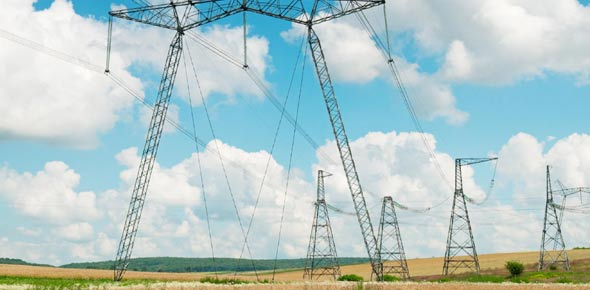# Block 1 Electricity Quiz

20 Questions | Total Attempts: 44Settings9-12. P. 3. 3 Students are able to describe electrical effects in terms of motion and concentrations of charged particles.

• 1.
How do like charges act when put near each other?
• A.

Attract

• B.

Repel or pull apart

• C.

Push together

• 2.
In a series circuit, electricity travels on ____________ path(s).
• A.

Three

• B.

Two

• C.

One

• D.

More than one

• 3.
Current that flows in one direction is called _________________________.
• A.

Electric

• B.

Direct current

• C.

Alternating current

• D.

Series current

• 4.
Most circuits in the school building are _______________ _________________.
• A.

Direct current

• B.

Series circuit

• C.

Parallel circuit

• 5.
In order for current to flow, what do you need to have the following:  (Select the correct answers)
• A.

Wire

• B.

Power source

• C.

Switch

• D.

Device to use the current ex. light

• 6.
If you take one light bulb out of a string of bulbs and the rest of them go out, you have what type of circuit.
• A.

Parallel circuit

• B.

Alternating current

• C.

Direct current

• D.

Series circuit

• 7.
A flashlight is example of what kind of circuit.
• A.

Parallel circuit

• B.

Series circuit

• 8.
What type of natural phenomena is an example of static electricity?
• A.

Hurricane

• B.

Lightning

• C.

• D.

Thunder

• 9.
Current that that flows on more than one path is called a _________________.
• A.

Alternating current

• B.

Series circuit

• C.

Direct current

• D.

Parallel circuit

• 10.
If you have a string of lights and you add another light, all of the lights become dimmer.  What type of circuit is this?
• A.

Parallel circuit

• B.

Series circuit

• C.

Direct current

• D.

Alternating current

• 11.
A diagram that uses symbols to show the parts of a circuit.
• A.

Conductor

• B.

Insulator

• C.

Schematic

• D.

Resistance

• 12.
A measure of how easily electric current will flow through a material.
• A.

Insulator

• B.

Conductor

• C.

Resistance

• D.

Alternating current

• 13.
The type of current in the school building is _________________ current.
• A.

Alternating

• B.

Direct

• C.

Series

• D.

Parallel

• 14.
Most circuits in homes or in the school building are ______________.
• A.

1000 volt

• B.

120 volts

• C.

120 watts

• D.

240 volts

• 15.
Choose the following items that are insulators.
• A.

Rubber

• B.

Copper

• C.

Plastic

• D.

Gold

• E.

Silver

• F.

Wood

• 16.
If a hair dryer uses 1.584 kw an hour and you use the hair dryer .5 hours a day, how much energy does the hair dryer use every day?
• A.

792 kwh

• B.

.792 kwh

• C.

79.2 kwh

• D.

7.92 kwh

• 17.
If a halogen lamp uses 12.6 kw of energy in a week, how much does the electricity cost for the lamp if electricity is \$.10 an a kWh?
• A.

\$126.00

• B.

126 kWh

• C.

\$12.60

• D.

\$1.26

• 18.
What helps prevent fires when a circuit is overloaded?
• A.

Circuit breaker

• B.

Wire

• C.

Switch

• 19.
A field in a region of space around a charge that shows fields of positive or negative charge
• A.

Electric charge

• B.

Electric force

• C.

Electric field

• D.

Electric current

• 20.
Earth is like a giant_______________.
• A.

Sphere

• B.

Magnet

• C.

Force field

• D.

Pole

Related TopicsBack to top